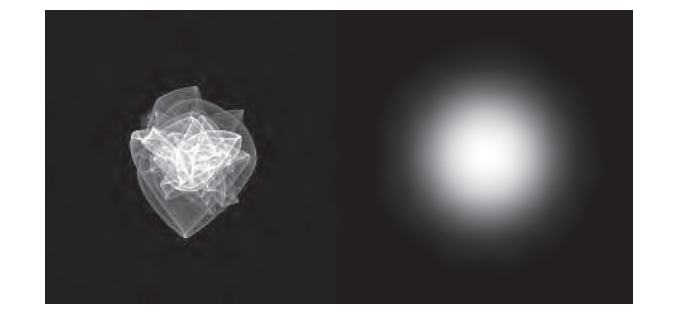"Let the form of an object be what it may,|light, shade, and
perspective will always make it beautiful. " — John Constable

### 9.1 Physics of Light（光物理）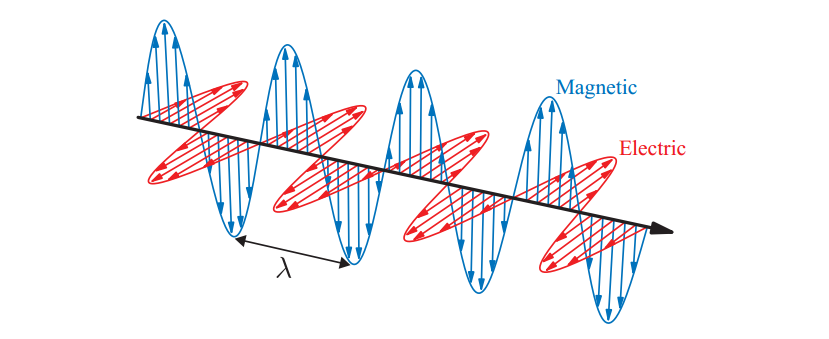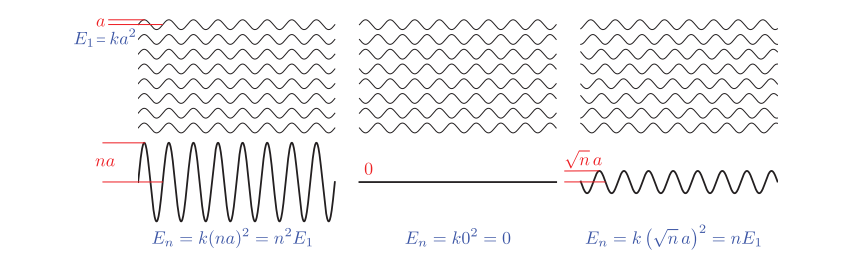#### 9.1.2 Media（介质）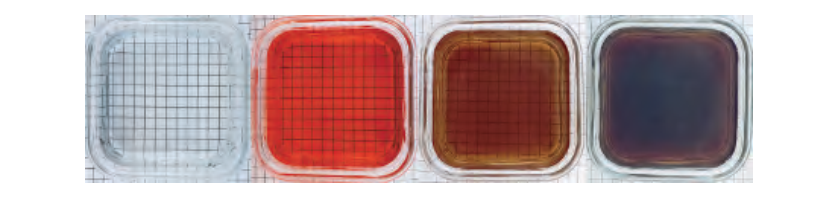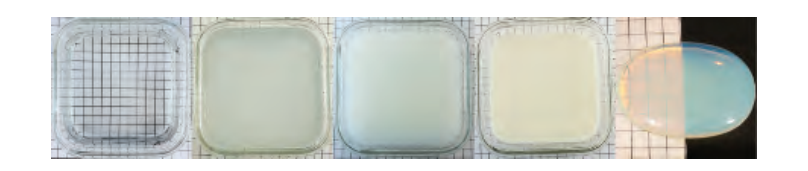#### 9.1.3 Surfaces（表面）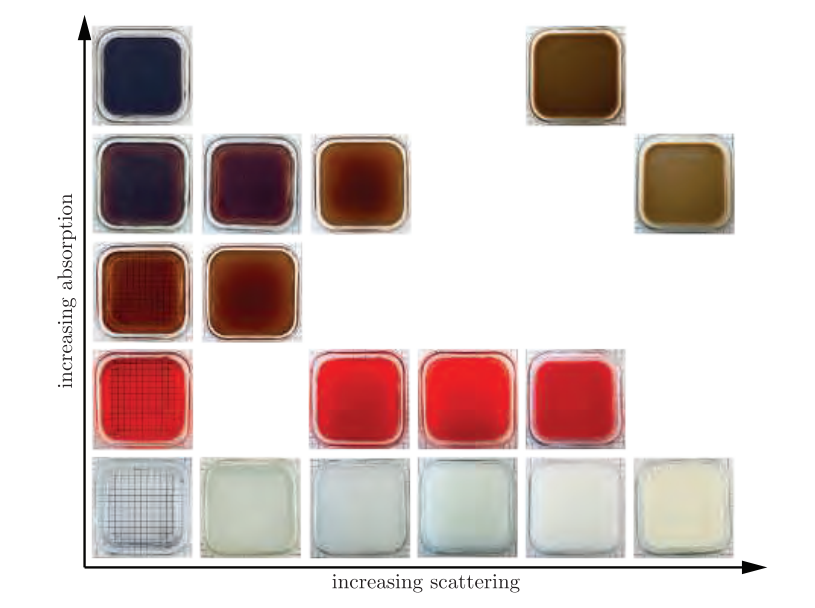1. 在表面，任何散射波必须与入射波同相或180°异相。因此，在表面处，散射波的峰值必须与入射波的峰值或波谷对齐。这限制了散射波仅沿两个可能的方向进入，一个向前延伸到表面，一个向远离它的方向。第一个是透射波（transmitted wave），第二个是反射波（reflected wave）。
2. 散射波必须与入射波具有相同的频率。我们在这里假设单色波，但我们讨论的原理可以通过首先将其分解为单色分量来应用于任何一般波。
3. 当光波从一种介质移动到另一种介质时，波通过介质的相速度与相对折射率$(n_1/n_2)$成比例地变化。 由于频率是固定的，因此波长也与$(n_1/n_2 )$成比例地变化。

$$\sin(\theta_t)=\frac{n_1}{n_2}\sin(\theta_i).\tag{9.1}$$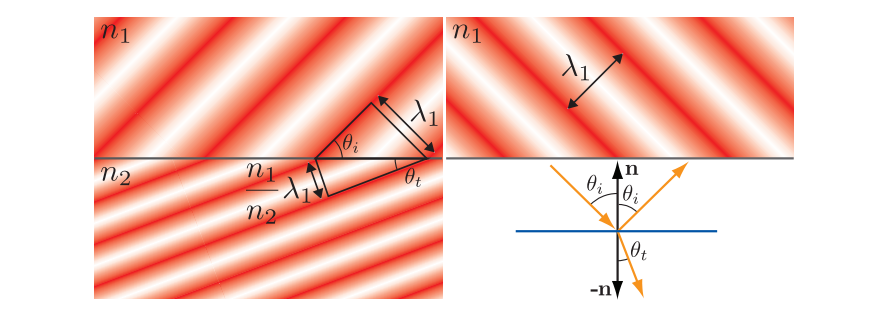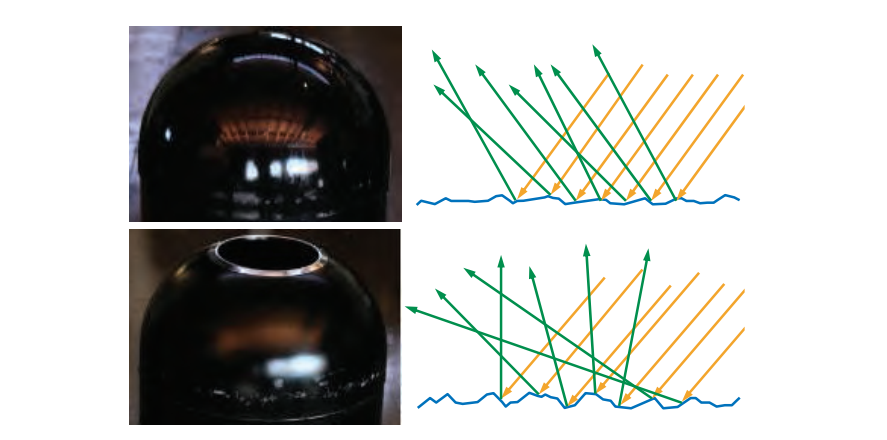#### 9.1.4 Subsurface Scattering（次表面散射）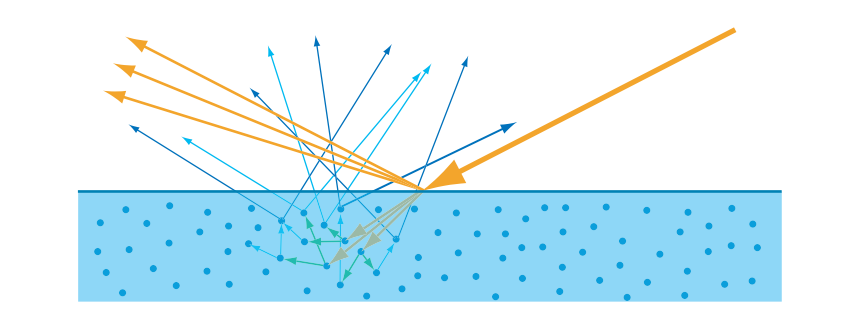### 9.2 The Camera（相机）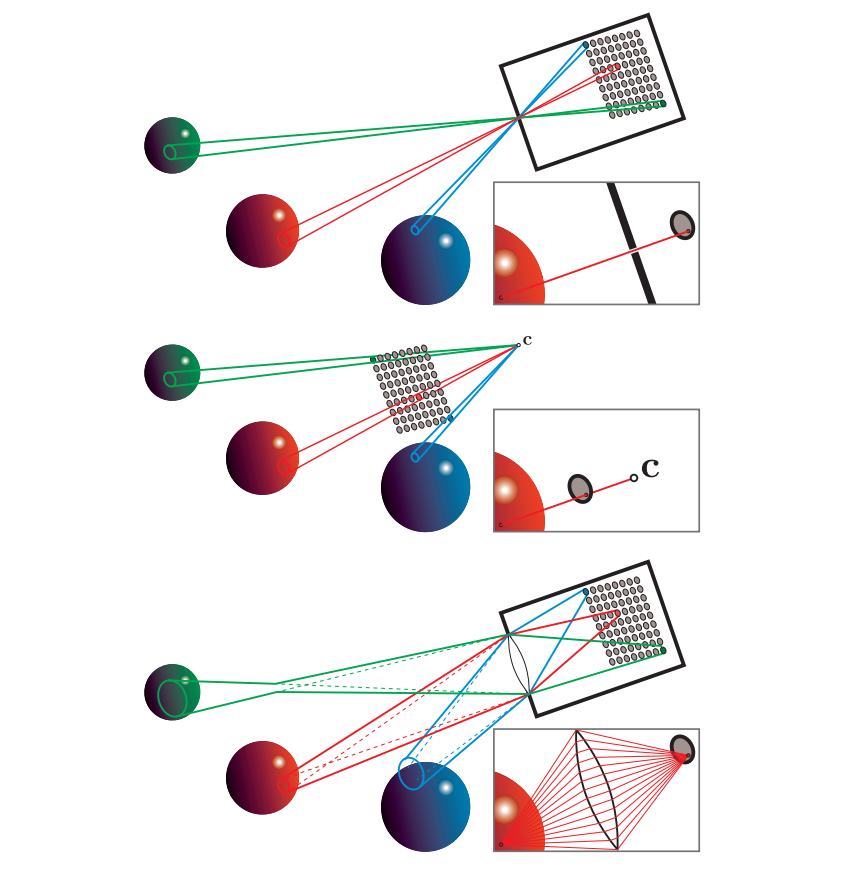### 9.3 The BRDF（BRDF）

$$L_i(\boldsymbol{c},\boldsymbol{-v})=L_o(\boldsymbol{p},\boldsymbol{v})\tag{9.2}$$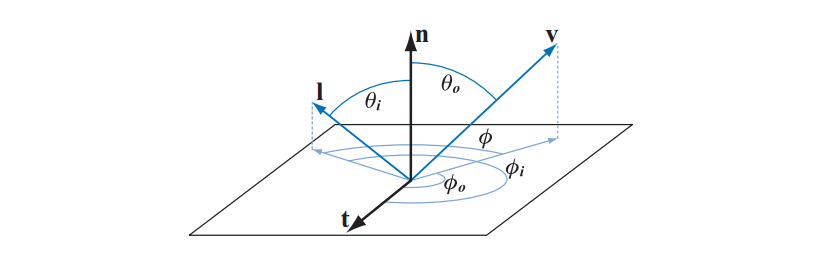$$L_o(\boldsymbol{p},\boldsymbol{v})=\int_{\boldsymbol{l}\in\Omega}f(\boldsymbol{l},\boldsymbol{v})L_i(\boldsymbol{p},\boldsymbol{l})(\boldsymbol{n}\cdot\boldsymbol{l})d\boldsymbol{l}.\tag{9.3}$$

$$L_o(\boldsymbol{v})=\int_{\boldsymbol{l}\in\Omega}f(\boldsymbol{l},\boldsymbol{v})L_i(\boldsymbol{l})(\boldsymbol{n}\cdot\boldsymbol{l})d\boldsymbol{l}.\tag{9.4}$$

$$L_o(\theta_o,\phi_o)=\int_{\phi_i=o}^{2\pi}\int_{\theta_i=0}^{\pi/2}f(\theta_i,\phi_i,\theta_o,\phi_o)L_i(\theta_i,\phi_i)\cos\theta_i\sin\theta_id\theta_id\phi_i.\tag{9.5}$$

$$L_o(\mu_o,\phi_o)=\int_{\phi_i=o}^{2\pi}\int_{\mu_i=0}^1f(\mu_i,\phi_i,\mu_o,\phi_o)L_i(\mu_i,\phi_i)\mu_id\mu_id\phi_i.\tag{9.6}$$

$$f(\boldsymbol{l},\boldsymbol{v})=f(\boldsymbol{v},\boldsymbol{l})\tag{9.7}$$

$$R(\boldsymbol{l})=\int_{\boldsymbol{v}\in\Omega}f(\boldsymbol{l},\boldsymbol{v})(\boldsymbol{n}\cdot\boldsymbol{v})d\boldsymbol{v}\tag{9.8}$$

$$R(\boldsymbol{v})=\int_{\boldsymbol{l}\in\Omega}f(\boldsymbol{l},\boldsymbol{v})(\boldsymbol{n}\cdot\boldsymbol{l})d\boldsymbol{l}\tag{9.9}$$

$$R(\boldsymbol{l})=\pi f(\boldsymbol{l},\boldsymbol{v})\tag{9.10}$$

Lambertian BRDF的恒定反射率值通常被称为漫反射色彩$c_{\text{diff}}$或反照率$\rho$。在本章中，为了强调与次表面散射的联系，我们将这个量称为次表面反照率（subsurface albedo）$\rho_{ss}$。 第9.9.1节详细讨论了次表面反照率。公式9.10中的BRDF给出以下结果：

$$f(\boldsymbol{l},\boldsymbol{v})=\frac{\rho_{ss}}{\pi}.\tag{9.11}$$

1/π是由于在半球上积分余弦产生π值引起的，这些因素常见于BRDF。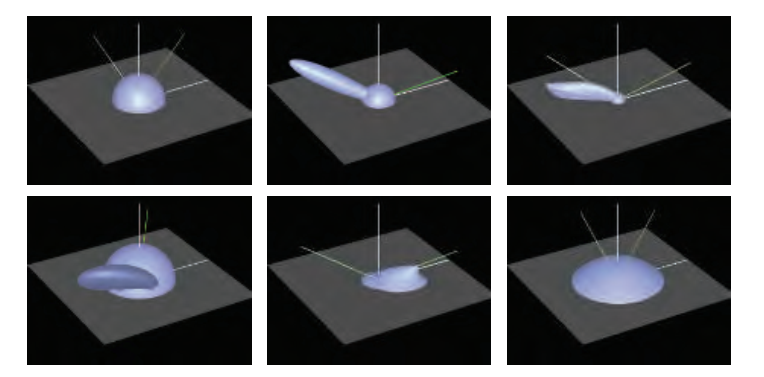### 9.4 Illumination（照明）

$$L_o(\boldsymbol{v})=\pi f(\boldsymbol{l}_c,\boldsymbol{v})\boldsymbol{c}_{light}\boldsymbol{n}\cdot\boldsymbol{l}_c)\tag{9.12}$$

$$L_o(\boldsymbol{v})=\pi f(\boldsymbol{l}_c,\boldsymbol{v})\boldsymbol{c}_{light}(\boldsymbol{n}\cdot\boldsymbol{l}_c)^+.\tag{9.13}$$

$$L_o(\boldsymbol{v})=\pi\sum^n_{i=1}f(\boldsymbol{l}_{c_i},\boldsymbol{v})\boldsymbol{c}_{light_{i}}(\boldsymbol{n}\cdot\boldsymbol{l}_{c_i})^+,\tag{9.14}$$

### 9.5 Fresnel Reflectance（菲涅尔反射）

$$\boldsymbol{r}_i=2(\boldsymbol{n}\cdot\boldsymbol{l})\boldsymbol{n}-\boldsymbol{l}.\tag{9.15}$$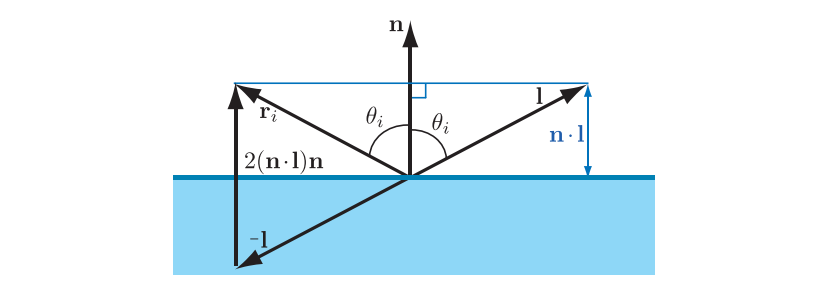#### 9.5.1 External Reflection（外反射）

External Reflection是n1 <n2的情况。 换句话说，光源自折射率较低的表面一侧。 大多数情况下，这一面含有空气，折射率约为1.003。 为简单起见，我们假设n1 = 1。 从物体到空气的相反过渡称为内反射（internal reflection），稍后将在9.5.3节中讨论。

• 当$\theta_i=0°$ 时，光垂直于表面$(l=n)$，$F(\theta_i)$具有作为物质属性的值。该值$F_0$可以被认为是物质的特征镜面颜色。$\theta_i= 0°$时的情况称为法向入射（normal incidence）。
• 当$\theta_i$ 增加并且光以越来越多的掠射角照射到表面时，$F(\theta_i)$的值将趋于增加，在$\theta_i= 90°$时所有频率值达到值1（白色）。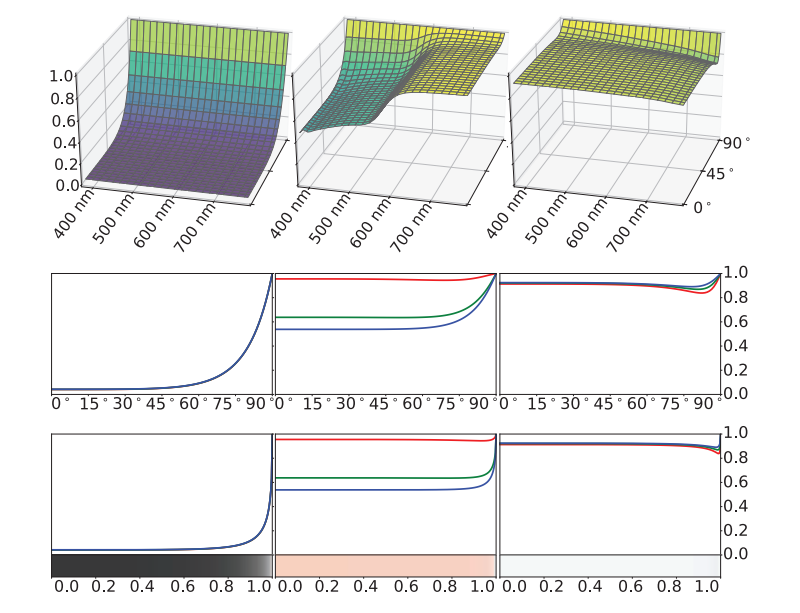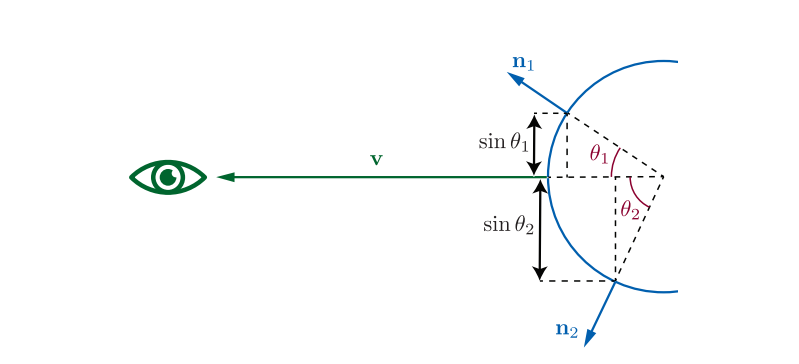$$F(\boldsymbol{n},\boldsymbol{l})\approx F_0+(1-F_0)(1-(\boldsymbol{n\cdot l})^+)^5.\tag{9.16}$$

$$F_0=(\frac{n-1}{n+1})^2\tag{9.17}$$

$$F(\boldsymbol{n},\boldsymbol{l})\approx F_0+(F_{90}-F_0)(1-(\boldsymbol{n\cdot l})^+)^{\frac1p}\tag{9.18}$$

#### 9.5.2 Typical Fresnel Reflectance Values（典型的菲涅尔反射值）

##### Fresnel Reflectance Values for Dielectrics （电介质的菲涅尔反射率）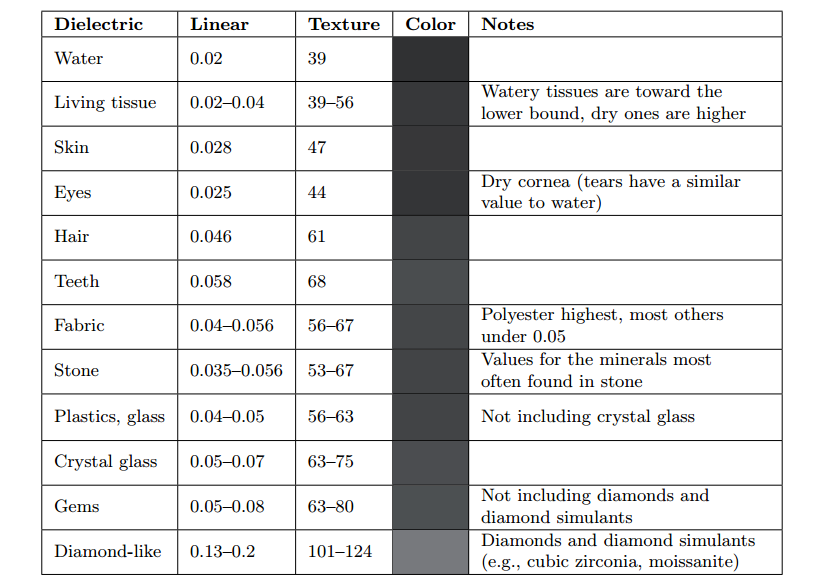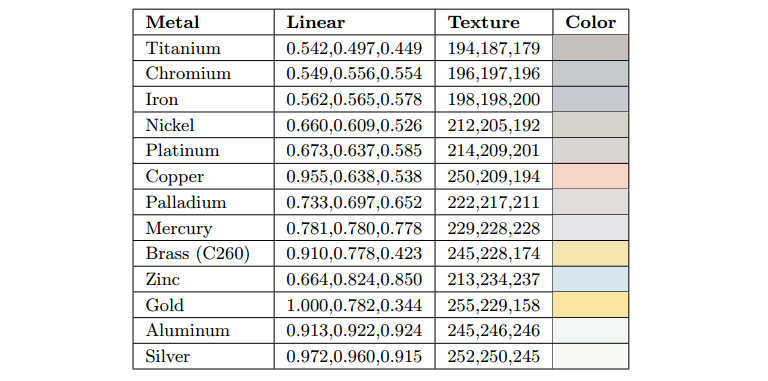##### Fresnel Reflectance Values for Semiconductors 半导体的菲涅尔反射值##### Fresnel Reflectance Values in Water

$$F_0=(\frac{n_1-n_2}{n_1+n_2})^2.\tag{9.19}$$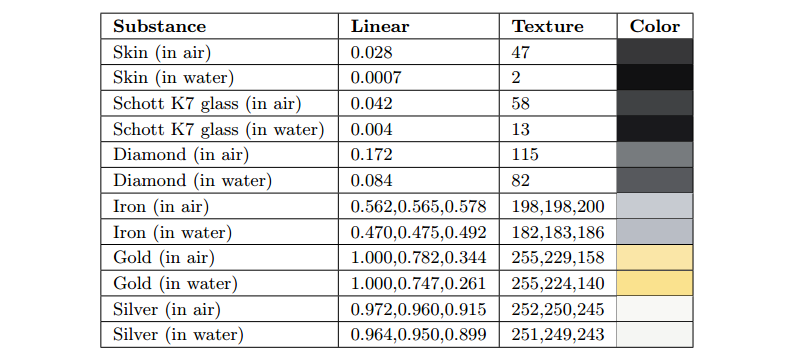#### 9.5.3 Internal Reflection（内反射）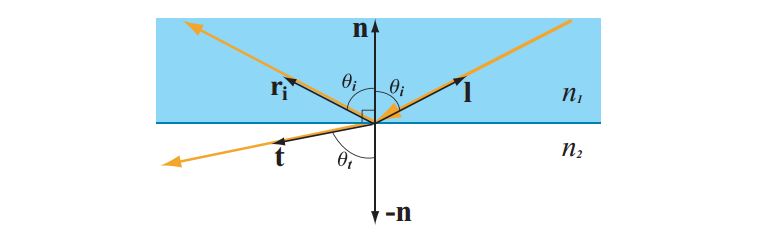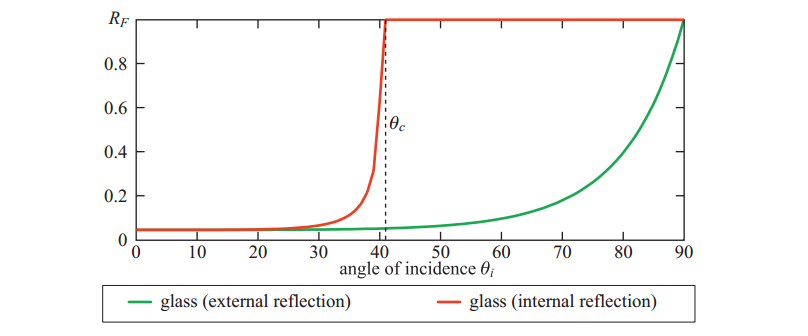$$\sin\theta_c=\frac{n_2}{n_1}=\frac{1-\sqrt{F_o}}{1+\sqrt{F_0}}.\tag{9.20}$$

### 9.6 Microgeometry（微观几何学）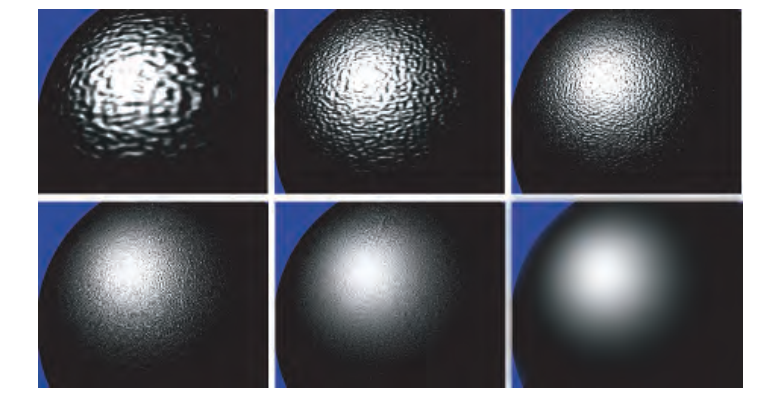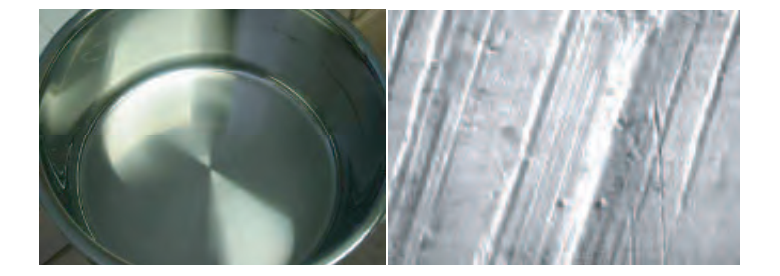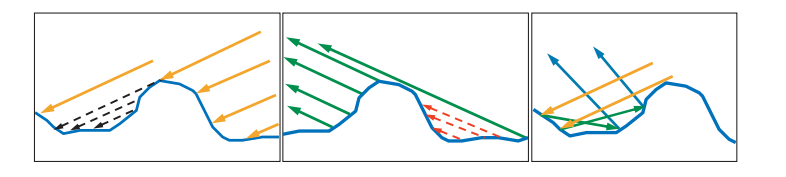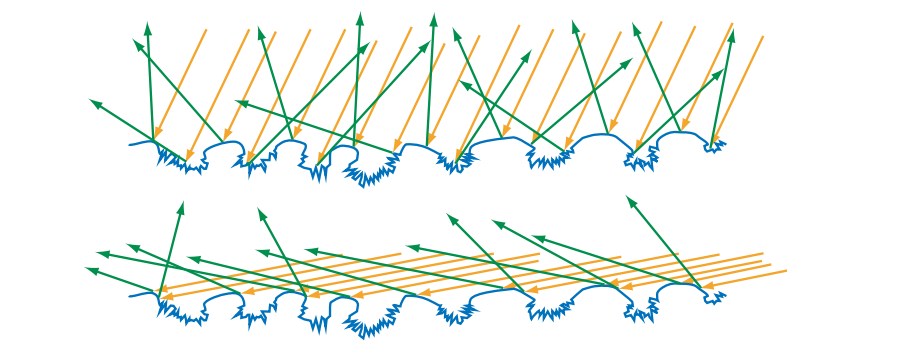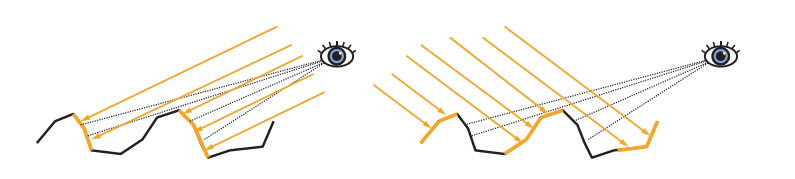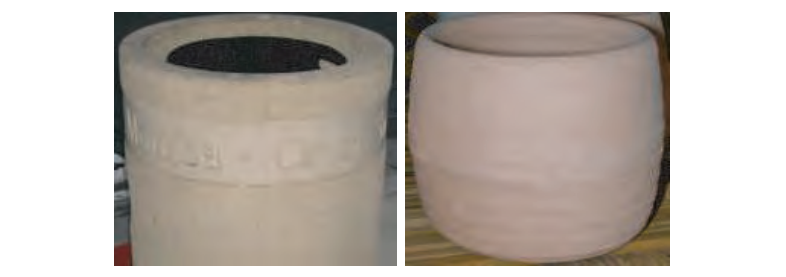### 9.7 Microfacet Theory（微面理论）

microfacet模型的一个重要特性是microfacet法线m的统计分布。此分布由曲面的正态分布函数或（normal distribution function）NDF定义。一些参考文献使用术语“（distribution of normals）法线分布”来避免与正态分布混淆。我们将使用$D(\boldsymbol{m})$来指代方程中的NDF。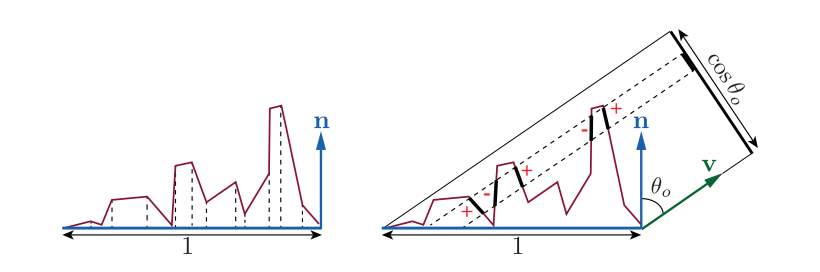NDF $D(\boldsymbol{m})$是微观几何表面面积上的微平面表面法线的统计分布。将$D(\boldsymbol{m})$积分在整个微平面法线上，得到微表面的面积。更有用的是，积分$D(\boldsymbol{m})(\boldsymbol{n·m})$，$D(\boldsymbol{m})$投影到宏观表面平面上，按照惯例给出宏观曲面贴片的面积等于1，如图9.31左侧所示。换句话说，投影$D(\boldsymbol{m})(\boldsymbol{n·m})$被归一化：

$$\int_{\boldsymbol{m}\in\Theta}D(\boldsymbol{m})(\boldsymbol{n·m})d\boldsymbol{m}=1\tag{9.21}$$

$$\int_{\boldsymbol{m}\in\Theta}D(\boldsymbol{m})(\boldsymbol{n·m})d\boldsymbol{m}=\boldsymbol{v\cdot n}.\tag{9.22}$$

$$\int_{\boldsymbol{m}\in\Theta}G_1(\boldsymbol{m,v})D(\boldsymbol{m})(\boldsymbol{v·m})^+d\boldsymbol{m}=\boldsymbol{v\cdot n}.\tag{9.23}$$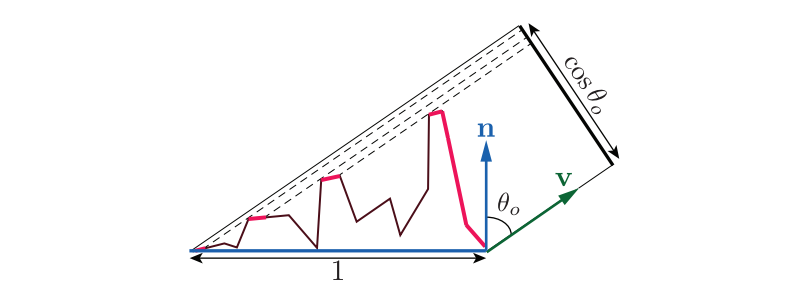$$G_1(\boldsymbol{m},\boldsymbol{v})=\frac{\chi^+(\boldsymbol{m\cdot v})}{1+\Lambda(\boldsymbol{v})},\tag{9.24}$$

$$\chi^+(x)= \begin{cases} 1,;where;x\gt0,\\ 0,;where;x\le0. \end{cases}\tag{9.25}$$

$$f(\boldsymbol{l},\boldsymbol{v})=\int_{\boldsymbol{m}\in\Omega}f_μ(\boldsymbol{l, v, m})G_2(\boldsymbol{l,v,m})D(\boldsymbol{m})\boldsymbol{\frac{(m\cdot l)^+}{\vert n\cdot l \vert}\frac{(m\cdot v)^+}{\vert n\cdot v \vert}}d\boldsymbol{m}.\tag{9.26}$$

Heitz讨论了$G_2$ 函数的几个版本。最简单的是可分离的形式，其中使用$G_1$ 分别评估掩蔽和阴影并相乘：

$$G_2(\boldsymbol{l,v,m})=G_1(\boldsymbol{v,m})G_1(\boldsymbol{l,m})\tag{9.27}$$

$$G_2(\boldsymbol{l,v,m})=\lambda(\phi)G_1(\boldsymbol{v,m})G_1(\boldsymbol{l,m})+(1-\lambda(\phi))min(G_1(\boldsymbol{v,m}),G_1(\boldsymbol{l,m})),\tag{9.28}$$

$$\lambda{(\phi)}=1-e^{-7.3\phi^2}.\tag{9.29}$$

van Ginneken等人提出了不同的λ函数：

$$\lambda(\phi)=\frac{4.41\phi}{4.41\phi+1}.\tag{9.30}$$

$$G_2(\boldsymbol{l,v,m})=\frac{\chi^+(\boldsymbol{m\cdot v})\chi^+(\boldsymbol{m\cdot l})}{1+\Lambda(\boldsymbol{v})+\Lambda(\boldsymbol{l})}.\tag{9.31}$$

Heitz还描述了一种结合方向和高度相关性的Smith $G_2$ 形式：

$$G_2(\boldsymbol{l,v,m})=\frac{\chi^+(\boldsymbol{m\cdot v})\chi^+(\boldsymbol{m\cdot l})}{1+max(\Lambda(\boldsymbol{v}),\Lambda(\boldsymbol{l}))+\lambda(\boldsymbol{v,l})min(\Lambda(\boldsymbol{v}),\Lambda(\boldsymbol{l}))},\tag{9.32}$$

### 9.8 BRDF Models for Surface Reflection（表面反射的BRDF模型）

$$\boldsymbol{h}=\frac{\boldsymbol{l+v}}{\Vert\boldsymbol{l+v}\Vert}.\tag{9.33}$$$$f_{spec}(\boldsymbol{l,v})=\frac{F(\boldsymbol{h,l})G_2(\boldsymbol{l,v,h})D(\boldsymbol{h})}{4\vert\boldsymbol{n\cdot l}\vert\vert\boldsymbol{n\cdot v}\vert}\tag{9.34}$$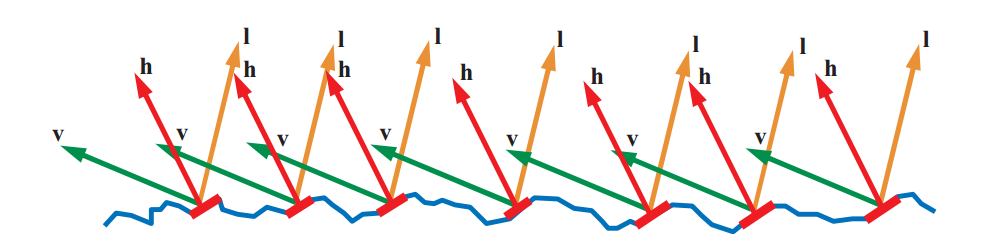#### 9.8.1 Normal Distribution Functions（法线分布函数）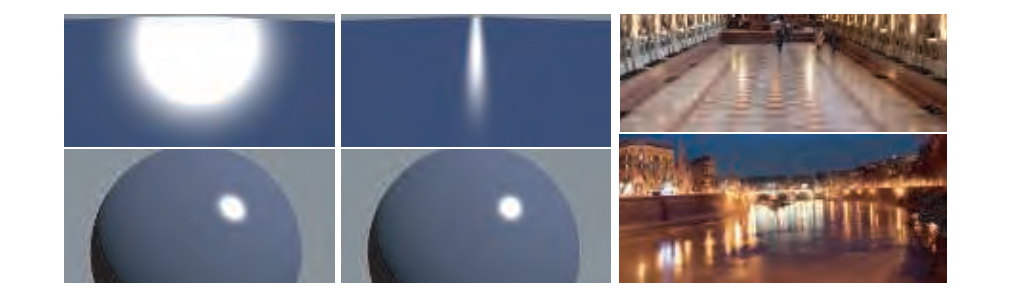##### Isotropic Normal Distribution Functions 各向同性的法线分布函数

Beckmann NDF是由光学界开发的第一批微面模型中使用的正态分布。它至今仍在该社区中广泛使用。它也是Cook-Torrance BRDF选择的NDF。归一化的Beckmann分布具有以下形式：

$$D(\boldsymbol{m})=\frac{\chi^+(\boldsymbol{n\cdot m})}{\pi\alpha^2_b(\boldsymbol{n\cdot m})^4}\exp(\frac{(\boldsymbol{n\cdot m})^2-1}{\alpha^2_b(\boldsymbol{n\cdot m})^2}).\tag{9.35}$$

Beckmann NDF是形状不变的（shape-invariant），它简化了$Λ$的推导。如Heitz所定义的，如果其粗糙度参数的效果等于缩放（拉伸）微表面，则各向同性NDF是形状不变的。形状不变的NDF可以用以下形式编写：

$$D(\boldsymbol{m})=\frac{\chi^+(\boldsymbol{n\cdot m})}{\pi\alpha^2_b(\boldsymbol{n\cdot m})^4}g(\frac{\sqrt{1-(\boldsymbol{n\cdot m})^2}}{\alpha(\boldsymbol{n\cdot m})}),\tag{9.36}$$

$$a=\frac{\boldsymbol{n\cdot s}}{\alpha\sqrt{1-(\boldsymbol{n\cdot s})^2}},\tag{9.37}$$

Beckmann NDF的$Λ$函数是：

$$\Lambda(a)=\frac{erf(a)-1}{2}+\frac1{2a\sqrt{\pi}}\exp(-a^2).\tag{9.38}$$

$$\Lambda(a)\approx \begin{cases} \frac{1-1.259a+0.396a^2}{3.535a+2.181a^2},&where;a\lt1.6,\\ 0,&where;a\ge1.6. \end{cases}\tag{9.39}$$

Blinn-Phong NDF由Blinn推导出来，作为（基于非物理的）Phong着色模型的修改：

$$D(\boldsymbol{m})=\chi^+(\boldsymbol{n\cdot m})\frac{\alpha_p+2}{2\pi}(\boldsymbol{n\cdot m})^{\alpha_p}.\tag{9.40}$$

Blinn-Phong NDF不是形状不变的，并且其$Λ$函数不存在分析形式。沃尔特等人。建议将贝克曼$Λ$函数与$α_p=2α_b^{-2}-2$参数等价结合使用。

GGX distribution是：

$$D(\boldsymbol{m})=\frac{\chi^+(\boldsymbol{n\cdot m})\alpha_g^2}{\pi(1+(\boldsymbol{n\cdot m})^2(\alpha^2_g-1))^2}.\tag{9.41}$$

GGX分布是形状不变的，其Λ函数相对简单：

$$\Lambda(a)=\frac{-1+\sqrt{1+\frac{1}{a^2}}}{2}.\tag{9.42}$$

$$\frac{G_2(\boldsymbol{l,v})}{4\vert\boldsymbol{n\cdot l\vert\vert}\boldsymbol{n\cdot v\vert}}\Longrightarrow\frac{0.5}{\mu_o\sqrt{\alpha^2+\mu_i(\mu_i-\alpha^2\mu_i)}+\mu_i\sqrt{\alpha^2+\mu_o(\mu_o-\alpha^2\mu_o)}}.\tag{9.43}$$

$$G_1(\boldsymbol{s})\approx\frac{2(\boldsymbol{n\cdot s})}{(\boldsymbol{n\cdot s})(2-\alpha)+\alpha},\tag{9.44}$$

$$\frac{G_2(\boldsymbol{l,v})}{4\vert\boldsymbol{n\cdot l\vert\vert}\boldsymbol{n\cdot v\vert}}\approx\frac{0.5}{lerp(2\vert\boldsymbol{n\cdot l}\vert\vert\boldsymbol{n\cdot v}\vert,\vert\boldsymbol{n\cdot l}\vert+\vert\boldsymbol{n\cdot v}\vert,\alpha)},\tag{9.45}$$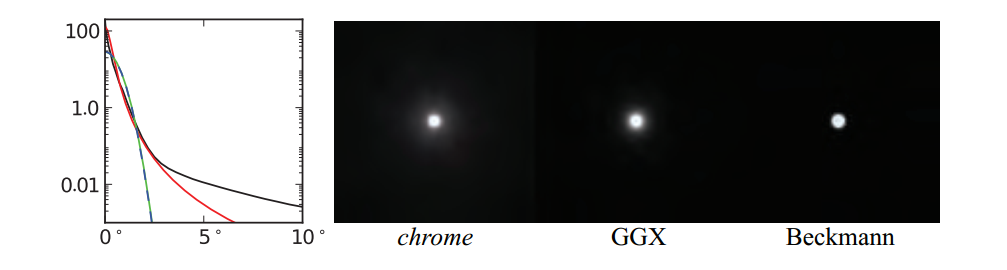Burley提出了广义的Trowbridge-Reitz（GTR）NDF，其目标是允许更多地控制NDF的形状，特别是分布的尾部：

$$D(\boldsymbol{m})=\frac{k(\alpha,r)}{\pi(1+(\boldsymbol{n\cdot m})^2(\alpha_g^2-1))^\gamma}.\tag{9.46}$$

γ参数控制尾部形状。当γ= 2时，GTR与GGX相同。随着γ的值减小，分布的尾部变得更长，并且随着它的增加，它们变得更短。在高γ值时，GTR分布类似于Beckmann。$k(α,γ)$项是归一化因子，我们在一个单独的方程中给出，因为它比其他NDF更复杂：

$$k(\alpha,\gamma)= \begin{cases} \frac{(\gamma-1)(\alpha^2-1)}{(1-(\alpha^2)^{(1-\gamma)})},&where;\gamma\ne1;and;\alpha\ne1,\\ \frac{\alpha^2-1}{\ln(\alpha^2)},&where;\gamma=1;and;\alpha\ne1,\\ 1,&where;\alpha=1. \end{cases}\tag{9.47}$$

GTR分布不是形状不变的，这使得找到其Smith G2掩蔽阴影函数变得复杂。NDF出版后花了三年时间才发布了$G_2$ 的解决方案。这个$G_2$ 解决方案非常复杂，有一些针对某些$γ$值的解析解表（对于中间值，必须使用插值）。GTR的另一个问题是参数α和γ以非直观的方式影响感知的粗糙度和"光韵"。

##### Anisotropic Normal Distribution Functions（各向异性的法线分布函数）

\begin{aligned}\boldsymbol{t'}=\boldsymbol{t}_0-(\boldsymbol{t_0\cdot\boldsymbol{n}})\boldsymbol{n}&\Longrightarrow\boldsymbol{t}=\frac{\boldsymbol{t'}}{\Vert\boldsymbol{t'}\Vert},\\ \left.\begin{array} \boldsymbol{\boldsymbol{b}}'=\boldsymbol{b_0-(b_0\cdot n)n},\\ \boldsymbol{b''=b'-(b'\cdot t)t}\\ \end{array} \right\rbrace &\Longrightarrow\boldsymbol{b=\frac{b''}{\Vert\boldsymbol{b''}\Vert}}. \end{aligned}\tag{9.48}

$$D(\boldsymbol{m})=\frac{\chi^+(\boldsymbol{n\cdot m})}{\alpha^2(\boldsymbol{n\cdot m})^4}g\left(\frac{\sqrt{1-(\boldsymbol{n\cdot m})^2}}{\alpha(\boldsymbol{n\cdot m})}\right),\tag{9.49}$$

g表示NDF形状的一维函数。各向异性版本是

$$D(\boldsymbol{m})=\frac{\chi^+(\boldsymbol{n\cdot m})}{\alpha_x\alpha_y(\boldsymbol{n\cdot m})^4}g\left(\frac{\sqrt{\frac{(\boldsymbol{t\cdot m})^2}{\alpha_x^2}+\frac{(\boldsymbol{b\cdot m})^2}{\alpha_y^2}}}{(\boldsymbol{n\cdot m})}\right),\tag{9.50}$$

$$a=\frac{\boldsymbol{n\cdot s}}{\sqrt{\alpha^2_x(\boldsymbol{t\cdot s})^2+\alpha_y^2(\boldsymbol{b\cdot s})^2}},\tag{9.51}$$

$$D(\boldsymbol{m})=\frac{\chi^+(\boldsymbol{n\cdot m})}{\pi\alpha_x\alpha_y(\boldsymbol{n\cdot m})^4}exp\left(-\frac{\frac{(\boldsymbol{t\cdot m})^2}{\alpha_x^2}+\frac{(\boldsymbol{b\cdot m})^2}{\alpha_y^2}}{(\boldsymbol{n\cdot m})^2}\right),\tag{9.52}$$

$$D(\boldsymbol{m})=\frac{\chi^+(\boldsymbol{n\cdot m})}{\pi\alpha_x\alpha_y(\frac{(\boldsymbol{t\cdot m})^2}{\alpha_x^2}+\frac{(\boldsymbol{b\cdot m})^2}{\alpha_y^2}+(\boldsymbol{n\cdot m})^2)^2}.\tag{9.53}$$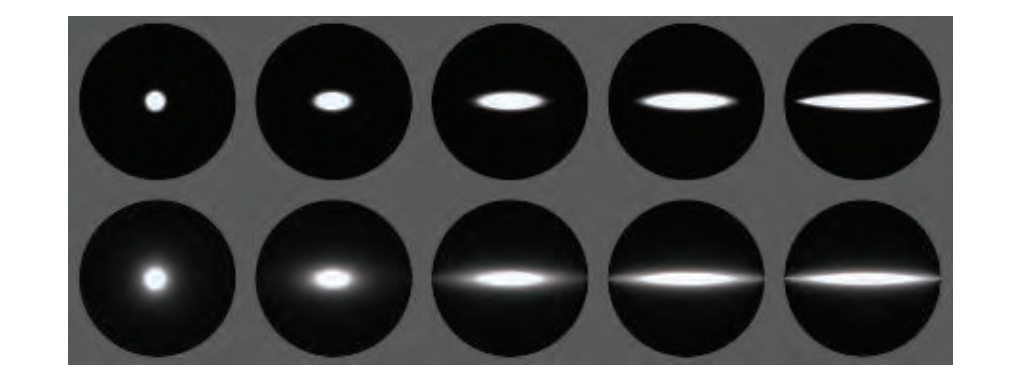\begin{align} k_{aspect}&=\sqrt{1-0.9k_{aniso}},\\ \alpha_x&=\frac{r^2}{k_{aspect}},\\ \alpha_y&=r^2k_{aspect}. \end{align}\tag{9.54}

0.9因子将纵横比限制为10:1。

Imageworks使用不同的参数化，允许任意程度的各向异性：

$$\alpha_x=r^2(1+k_{aniso}),\\ \alpha_y=r^2(1-k_{aniso}).\tag{9.55}$$

#### 9.8.2 Multiple-Bounce Surface Reflection（多次反射的表面反射）

Imageworks使用的一种技术结合了以前工作中的元素来创建一个可以添加到BRDF的公式，以模拟多次反射表面反射：

$$f_{ms}(\boldsymbol{l,v})=\frac{\overline{F}\overline{R_{sF1}}}{\pi(1-\overline{R_{sF1}})(1-\overline{F}(1-\overline{R_{sF1}}))}(1-R_{sF1}(\boldsymbol{l}))(1-R_{sF1}(\boldsymbol{v})),\tag{9.56}$$

\begin{align} \overline{R_{sF1}}&=\frac{\int_{s\in\Omega}R_{sF1}(s)(\boldsymbol{n\cdot s})ds}{\int_{s\in\Omega}R_{sF1}(\mu)\mu d\mu}=\frac1\pi\int_{\phi=0}^{2\pi}\int_{\mu=0}^{1}R_{sF1}(\mu)\mu d\mu d\phi \\ &=2\int^1_{\mu=0}R_{sF1}(\mu)\mu d\mu. \end{align}\tag{9.57}

$$\overline{F}=2\int^1_{\mu=0}F(\mu)\mu d\mu.\tag{9.58}$$

$$\overline{F}=\frac{2p^2F_{90}+(3p+1)F_0}{2p^2+3p+1}.\tag{9.59}$$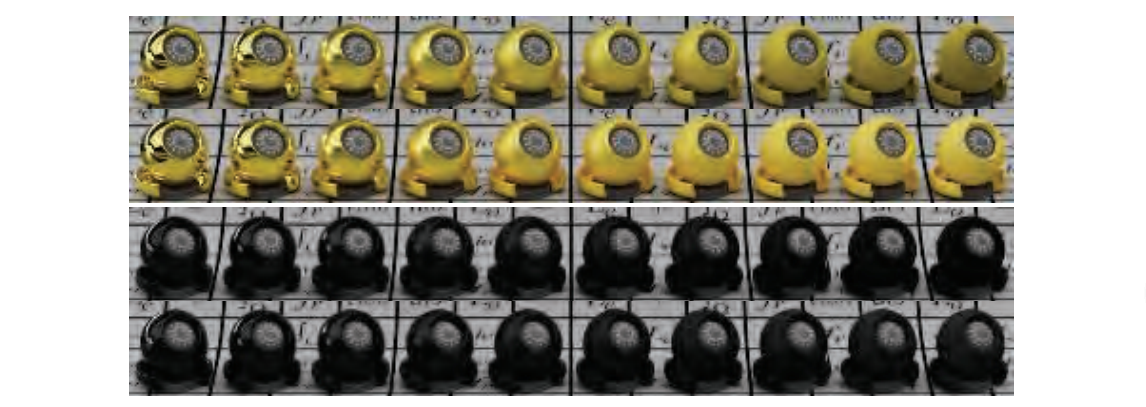$$\overline{F}=\frac{20}{21}F_0+\frac1{21}.\tag{9.60}$$

### 9.9 BRDF Models for Subsurface Scattering（次表面散射的BRDF模型）

#### 9.9.2 Scale of Subsurface Scattering and Roughness（次表面散射和粗糙度的比例）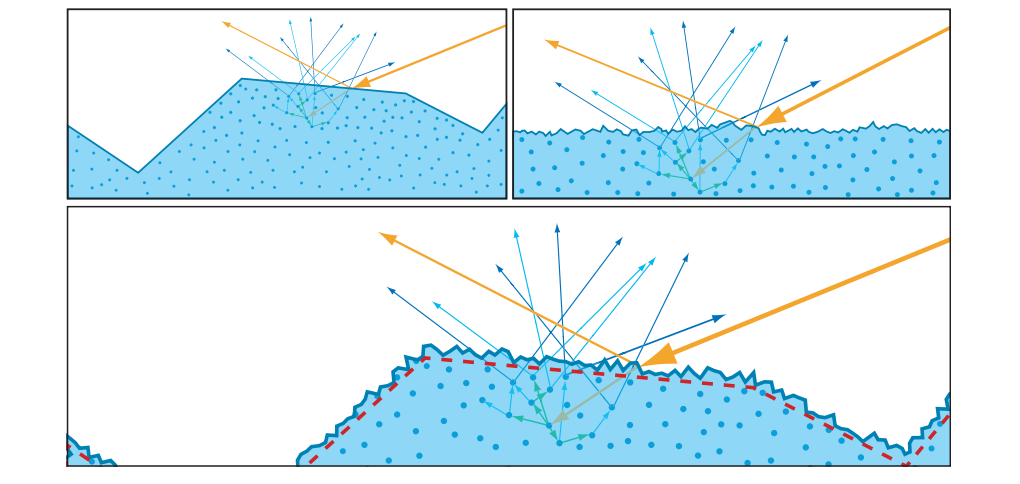#### 9.9.3 Smooth-Surface Subsurface Models（光滑表面的次表面模型）

$$f_{diff}(\boldsymbol{l,v})=\frac{\rho_{ss}}{\pi}.\tag{9.61}$$

Lambertian模型没有考虑到在表面反射的光不能用于次表面散射的事实。为了改进该模型，应该在表面（镜面）和次表面（漫反射）反射项之间进行能量权衡。菲涅耳效应意味着这种表面 - 次表面能量权衡随入射光角度θi而变化。 随着入射角越来越大，漫反射率随着镜面反射率的增加而降低。 解释这种平衡的一种基本方法是将漫反射项乘以一个减去镜面反射项的菲涅耳部分。 如果镜面术语是平面镜子，则得到的漫反射公式是：

$$f_{diff}(\boldsymbol{l,v})=(1-F(\boldsymbol{n,l}))\frac{\rho_{ss}}{\pi}.\tag{9.62}$$

$$f_{diff}(\boldsymbol{l,v})=(1-F(\boldsymbol{h,l}))\frac{\rho_{ss}}{\pi}\tag{9.63}$$

Shirley等人提出了平面耦合漫射项，解决了菲涅耳效应和表面 - 次表面反射率的权衡，同时支持节能和亥姆霍兹互易性。推导假设Schlick近似（公式9.16）用于菲涅耳反射：

$$f_{diff}(\boldsymbol{l,v})=\frac{21}{20\pi}(1-F_0)\rho_{ss}(1-(1-(\boldsymbol{n\cdot l})^+)^5)(1- (1-(\boldsymbol{n\cdot v})^+)^5)\tag{9.64}$$

$$f_{diff}(\boldsymbol{l,v})=\rho_{ss}\frac{(1-R_{spec}(\boldsymbol{l}))(1-R_{spec}(\boldsymbol{v}))}{\pi(1-\overline{R_{spec}})}.\tag{9.65}$$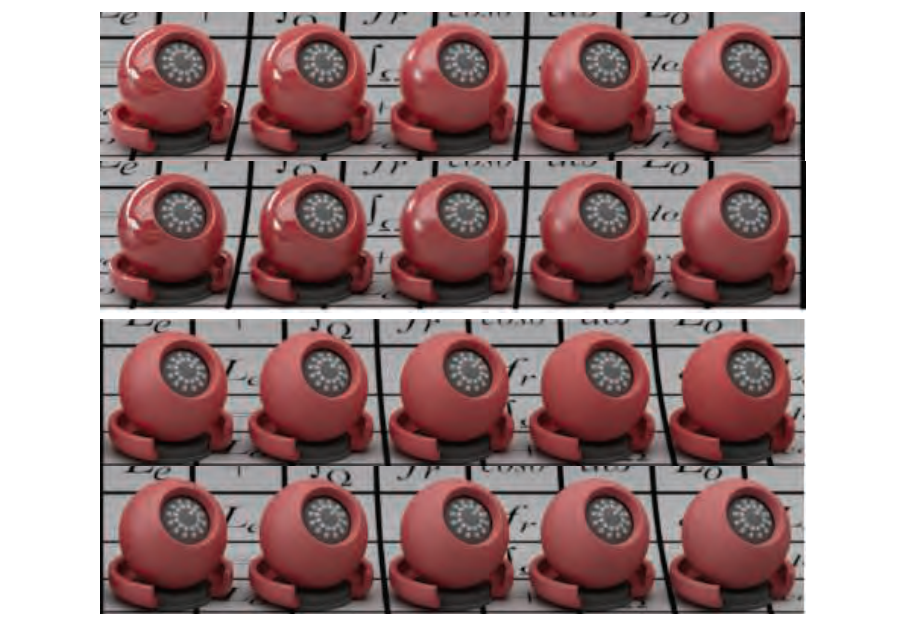#### 9.9.4 Rough-Surface Subsurface Models（粗糙表面次表面模型）

$$f_{diff}(\boldsymbol{l,v})=\chi^+(\boldsymbol{n\cdot l})\chi^+(\boldsymbol{n\cdot v})\frac{\rho_{ss}}{\pi}((1-k_{ss})f_d+1.25k_{ss}f_{ss}),\tag{9.66}$$

\begin{align} f_d&=(1+(F_{D90}-1)(1-\boldsymbol{n\cdot l})^5)(1+(F_{D90}-1)(1-\boldsymbol{n\cdot v})^5),\\ F_{D90}&=0.5+2\sqrt{\alpha}(\boldsymbol{h\cdot l})^2,\\ f_{ss}&=(\frac1{(\boldsymbol{n\cdot l})(\boldsymbol{n\cdot v})}-0.5)F_{ss}+0.5,\\ F_{ss}&=(1+(F_{SS90}-1))(1-\boldsymbol{n\cdot l})^5)(1+(F_{SS90}-1)(1-\boldsymbol{n\cdot v})^5),\\ F_{SS90}&=\sqrt\alpha(\boldsymbol{h\cdot l})^2, \end{align}\tag{9.67}

α是镜面粗糙度。 在各向异性的情况下，使用$α_x$ 和$α_y$ 之间的中间值。这个等式通常被称为迪斯尼漫射模型。

Oren-Nayar模型假设一个微观曲面具有完全不同的正态分布和遮蔽阴影函数，而不是当前镜面模型中使用的那些。 使用各向同性GGX NDF和高度相关的Smith掩蔽阴影函数导出两个漫射微平面模型。 Gotanda的第一个模型是数值积分一般微平面方程（公式9.26）的结果，使用公式9.64中的镜面耦合漫反射项作为微观BRDF。然后将分析函数拟合到数值积分数据中。Gotanda的BRDF没有考虑构面之间的相互反射，拟合函数相对复杂。

$$f_{diff}(\boldsymbol{l,v})=\chi^+(\boldsymbol{n\cdot l})\chi^+(\boldsymbol{n\cdot v})\frac{\rho_{ss}}{\pi}((1-\alpha_g)f_{smooth}+\alpha_gf_{rough}+\rho_{ss}f_{multi}),\tag{9.68}$$

\begin{align} f_{smooth}&=\frac{21}{20}(1-F_0)(1-(1-\boldsymbol{n\cdot l})^5)(1-(1-\boldsymbol{n\cdot v})^5),\\ f_{rough}&=k_{facing}(0.9-0.4k_{facing})(\frac{0.5+\boldsymbol{n\cdot h}}{\boldsymbol{n\cdot h}}),\\ k_{facing}&=0.5+0.5(\boldsymbol{l\cdot v}),\\ f_{multi}&=0.3641\alpha_g, \end{align}\tag{9.69}

$α_g$ 是GGX镜面粗糙度。为清楚起见，此处的公式与Hammon的ppt略有不同。注意，$f_{smooth}$是来自公式9.64的耦合漫射BRDF，没有$ρ_{ss}/π$因子，因为它在公式9.68中相乘。Hammon讨论了“混合”BRDF，它可替代其他平滑表面漫射BRDF，以提高性能或改善与旧模型下创作的资产的兼容性。

### 9.10 BRDF Models for Cloth（布料的BRDF模型）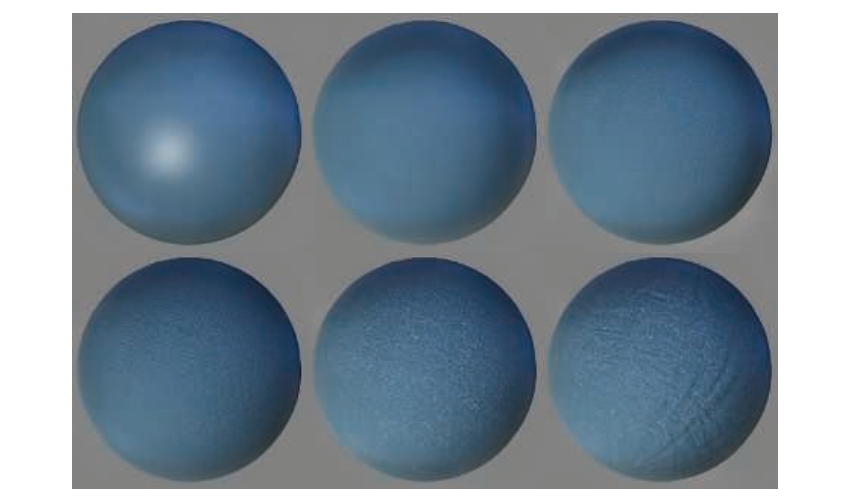#### 9.10.1 Empirical Cloth Models（经验布料模型）

$$f_{diff}(\boldsymbol{l,v})=\frac{\rho_{ss}}{\pi}(k_{rim}((\boldsymbol{v\cdot n})^+)^{\alpha_{rim}}+k_{inner}(1-(\boldsymbol{v\cdot n})^+)^{\alpha_{inner}}+k_{diff}),\tag{9.70}$$

$$f_{diff}(\boldsymbol{l,v})(\boldsymbol{n\cdot l})^+\Rightarrow\frac{\rho_{ss}}{\pi}(\boldsymbol{c}_{scatter}+(\boldsymbol{n\cdot l})^+)^{\mp}\frac{(\boldsymbol{n\cdot l}+w)^\mp}{1+w}.\tag{9.71}$$

$$f_{sheen}(\boldsymbol{l,v})=k_{sheen}\boldsymbol{c}_{sheen}(1-(\boldsymbol{h\cdot l})^+)^5,\tag{9.72}$$

#### 9.10.2 Microfacet Cloth Models（基于微面的布料模型）

$$D(\boldsymbol{m})=\frac{\chi^+(\boldsymbol{n\cdot m})}{\pi(1+k_{amp}\alpha^2)} \left( 1+\frac{k_{amp}exp(\frac{(\boldsymbol{n\cdot m})^2}{\alpha^2((\boldsymbol{n\cdot m})^2-1)})}{(1-(\boldsymbol{n\cdot m})^2)^2} \right),\tag{9.73}$$

$$f(\boldsymbol{l,v})=(1-F(\boldsymbol{h,l}))\frac{\rho_{ss}}{\pi}+\frac{F(\boldsymbol{h,l})D(\boldsymbol{h})}{4(\boldsymbol{n\cdot l}+\boldsymbol{n\cdot v}-(\boldsymbol{n\cdot l})(\boldsymbol{n\cdot v}))}.\tag{9.74}$$

Imageworks使用不同的反向NDF作为可以添加到任何BRDF的光泽项：

$$D(\boldsymbol{m})=\frac{\chi^+(\boldsymbol{n\cdot m})(2+\frac1\alpha)(1-(\boldsymbol{n\cdot m})^2)^{\frac1{2\alpha}}}{2\pi}.\tag{9.75}$$#### 9.10.3 Micro-Cylinder Cloth Models（微圆柱布料模型）

Dreamworks使用相对简单且可由艺术家控制的微圆柱模型用于织物。纹理可用于改变粗糙度，颜色和线的方向，这可以指出表面平面，用于模制天鹅绒和类似的织物。可以为经线和纬线设置不同的参数，以模拟复杂的变色织物，例如闪光的绸缎。为了节能，该模型被标准化。

### 9.11 Wave Optics BRDF Models（波光学的BRDF模型）

#### 9.11.1 Diffraction Models（衍射模型）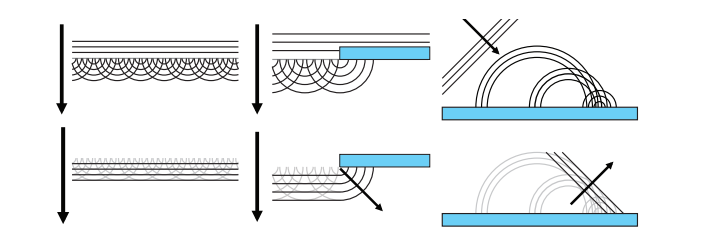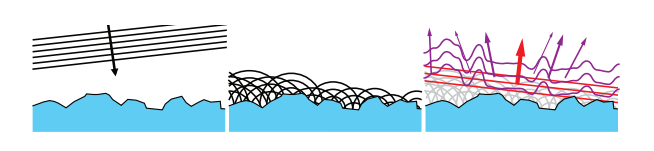#### 9.11.2 Models for Thin-Film Interference（薄膜干涉模型）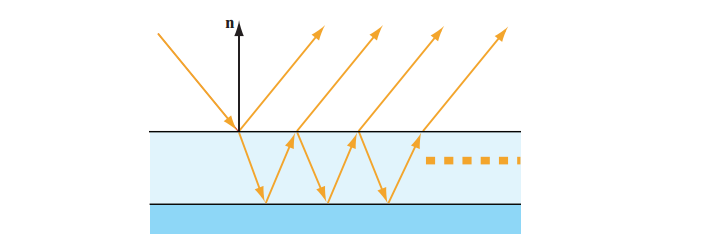### 9.12 Layered Materials（分层材质）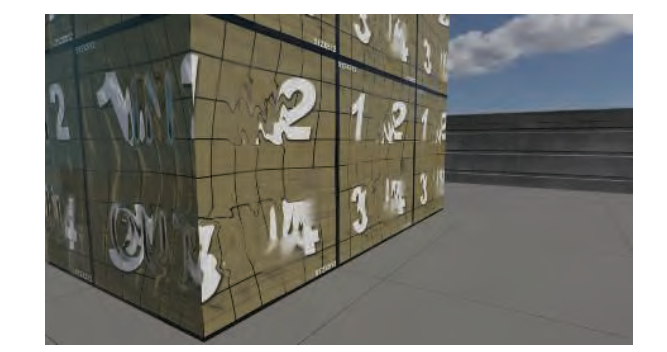### 9.13 Blending and Filtering Materials（混合和过滤材质）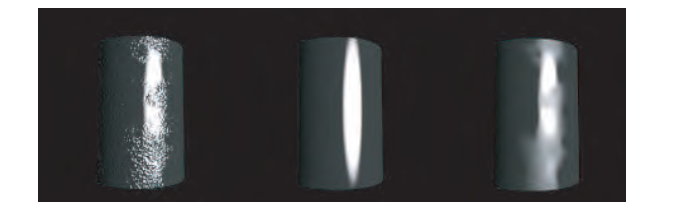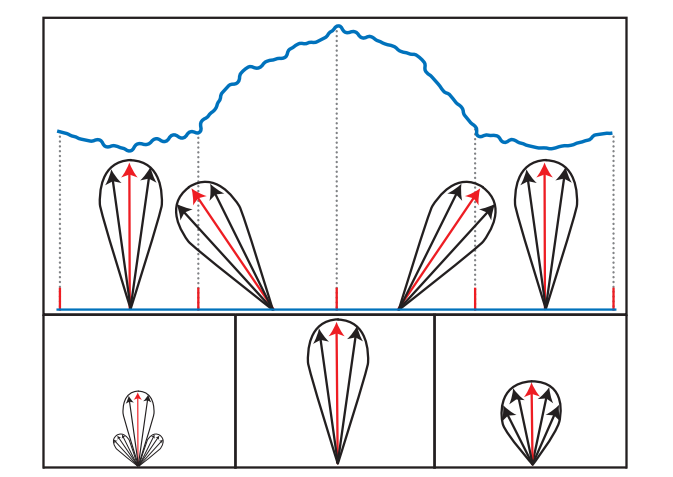Toksvig的原始方程旨在与Blinn-Phong NDF一起使用：

$$\alpha'_p=\frac{\Vert\overline{\boldsymbol{n}}\Vert\alpha_p}{\Vert\overline{\boldsymbol{n}}\Vert+\alpha_p(1-\Vert\overline{\boldsymbol{n}}\Vert)},\tag{9.76}$$

Toksvig的方法具有考虑GPU纹理过滤引入的正常方差的优点。它也适用于最简单的正常mipmapping方案，线性平均而不规范化。此功能对于动态生成的法线贴图尤其有用，例如水波纹，必须在运行时生成mipmap。 该方法不适用于静态法线贴图，因为它不能很好地适用于压缩法线贴图的主流方法。 这些压缩方法依赖于单位长度的正常情况。 由于Toksvig的方法依赖于平均法线变化的长度，因此与其一起使用的法线贴图可能必须保持未压缩。 即使这样，存储缩短的法线也会导致精度问题。